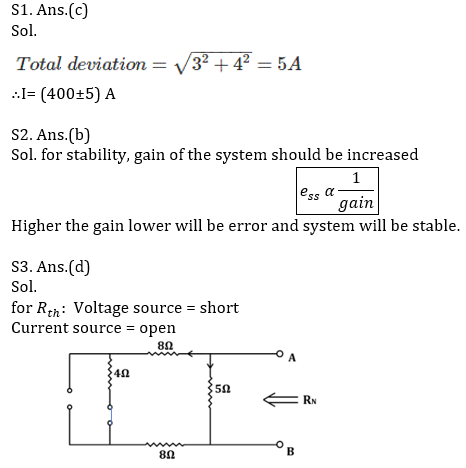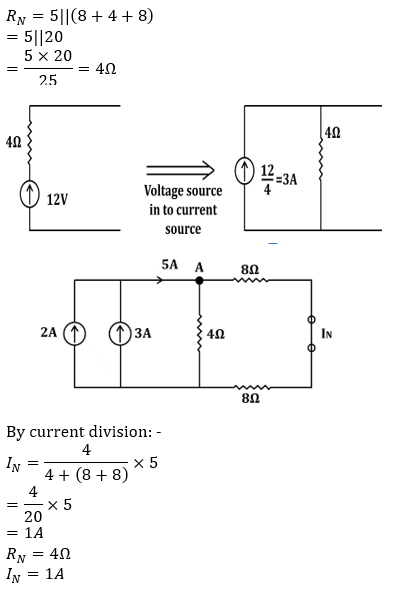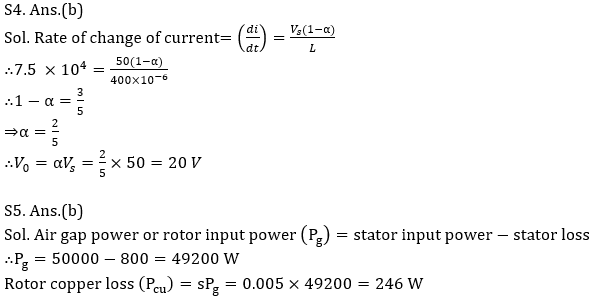Engineering Jobs   »   ELECTRICAL GATE QUIZ,

# GATE’22 EE: Daily Practices Quiz 26-July-2021

Each question 2 marks.
Negative marking: 0.66 mark

Total Questions: 05
Total marks: 10
Time: 12 min.

Q1. The total current I = I1 + I2 in a circuit is measured I1 = 150 ± 3A, I2 = 250 ± 4 A, where the limits of error are given as standard deviations. I measured as:
(a) (400 ± 3) A
(b) (400 ± 2.24) A
(c) (400 ±5) A
(d) (400 ± 1) A

Q2. Which of the following should be done to make an unstable system stable?
(a) The gain of the system should be decreased.
(b) The gain of the system should be increased.
(c) The number of poles to the loop transfer function should be increased.
(d) The number of zeros to the loop transfer function should be increased.

Q3. Find Norton equivalent resistance, R_N, and equivalent current source I_N at terminals A and B of the circuit.(a) R_N = 20Ω, I_N = 2A
(b) R_N = 20Ω, I_N = 1A
(c) R_N = 4Ω, I_N = 2A
(d) R_N = 4Ω, I_N = 1A

Q4. In a dc-dc buck converter when the rate of change of current in the 400 µH inductor, L is 7.5×10^4 A/sec. If the converter is operated with a 50 V input voltage, the output voltage of the converter is:
(a) 25 V
(b) 20 V
(c) 15 V
(d) 30 V

Q5. The power input to a 3-phase induction motor is 50 KW. If the stator losses are 800 W, then copper losses of rotor phase for 0.5 % slip will be:
(a) 492 W
(b) 246 W
(c) 200 W
(d) 100 W

SOLUTIONSSharing is caring!